Courses

# EMPIRICAL AND MOLECULAR FORMULAS Class 11 Notes | EduRev

## Class 11 : EMPIRICAL AND MOLECULAR FORMULAS Class 11 Notes | EduRev

The document EMPIRICAL AND MOLECULAR FORMULAS Class 11 Notes | EduRev is a part of Class 11 category.
All you need of Class 11 at this link: Class 11

EMPIRICAL FORMULAS
The Empirical formulas express the simplest atomic ratio between the elements in the compound. Such formulas are called empirical formulas. An empirical formula does not necessarily represent the actual numbers of atoms present in a molecule of a compound; it represents only the ratio between those numbers.
So it may or may not represent the actual formula of the molecule. It can be deduced by knowing the weight % of all the constituent element with their atomic masses for the given compound.
For example: C6H12O6, CH3COOH, HCHO
All have same empirical formula CH2O, but they are different.
The empirical formula of a compound can be determined by the following steps:

• Write the name of detected elements in column-1 present in the compound.
• Write the corresponding atomic mass in column-2.
• Write the experimentally determined percentage composition by weight of each element present in the compound in column-3.
• Divide the percentage of each element by its atomic weight to get the relative number of atoms of each element in column-4.
• Divide each number obtained for the respective elements in step (3) by the smallest number among those numbers so as to get the simplest ratio in column-5.
• If any number obtained in step (4) is not a whole number then multiply all the numbers by a suitable integer to get whole number ratio. This ratio will be the simplest ratio of the atoms of different elements present in the compound. Empirical formula of the compound can be written with the help of this ratio in column-6.

Example.1. A compound contains C = 71.23%, H = 12.95% and O = 15.81%. What is the empirical formula of the compound?
Solution.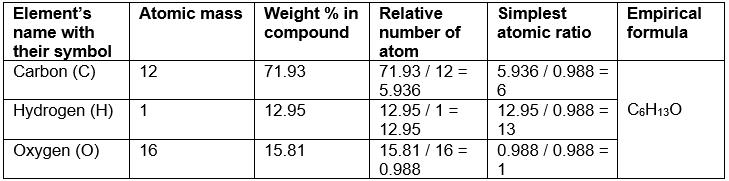Example.2. The simplest formula of a compound containing 50% of element X (Atomic mass = 10) and 50% of the element Y (Atomic mass = 20) is:
(A) XY
(B) X2Y

(C) XY2
(D) X2Y3
Ans. (b)
Solution.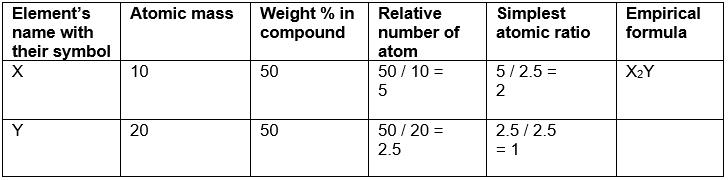MOLECULAR FORMULAS
The actual numbers of atoms of each element that occur in the smallest freely existing unit or molecule of the compound is expressed by the molecular formula of the compound.
The molecular formula of a compound may be the empirical formula, or it may be a multiple of the empirical formula.
Conclusively, The formula which represents the actual number of each individual atom in any molecule is known as molecular formula.

Molecular formula = (Empirical formula)n
Molecular weight = Empirical formula weight x n

If the vapour density of the substance is known, its molecular weight can be calculated by using the equation.
2 x vapour density = Molecular weight
For example, the molecular formula of butene, C4H8, shows that each freely existing molecule of butene contains four atoms of carbon and eight atoms of hydrogen. Its empirical formula is CH2.
One molecule of ethylene (molecular formula C2H4) contains two atoms of carbon and four atoms of hydrogen. Its empirical formula is CH2. Both have the same empirical formula, yet they are different compounds with different molecular formulas.
Butene is C4H8, or four times the empirical formula; ethylene is C2H4, or twice the empirical formula.

For certain compounds the molecular formula and the empirical formula may be same.

EXAMPLE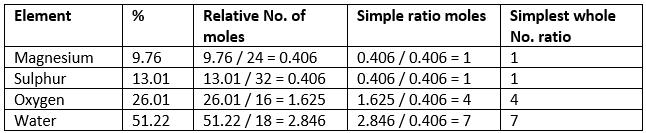Hence the empirical formula is Mg SO4.7H2O.

For Writing molecular formula
(a)Determine empirical formula mass Add the atomic masses of various atoms present in the empirical formula.
(b) Divide Molar mass by empirical formula mass which will give the ‘n’ factor
(c) Molecular formula = n X empirical formula

Try Yourself!
A compound contains 4.07 % hydrogen, 24.27 % carbon and 71.65 % chlorine. Its molar mass is 98.96 g. What are its empirical and molecular formulas ?

Example.3. A compound of carbon, hydrogen and nitrogen contains these elements in the ratio 9 : 1 : 3.5. Calculate the empirical formula. If its molecular mass is 108, what is the molecular formula?
Solution.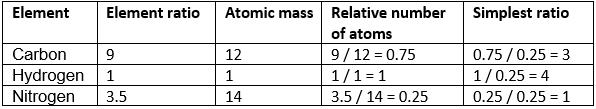Empirical formula = C3H4N
Empirical formula mass = (3 × 12) + (4 × 1) + 14
= 54

n = mol. mass / Emp. mass
= 108 / 54 = 2
Thus, molecular formula of the compound
= 2 × empirical formula

= 2 × C3H4N

= C6H8N2

Example.4. 2.38 gm of uranium was heated strongly in a current of air. The resulting oxide weighed 2.806 g. Determine the empirical formula of the oxide. (At. mass U = 238; O = 16).
Solution. Step 1: To calculate the percentage of uranium and oxygen in the oxide.
2.806 g of the oxide contain uranium = 2.38 g
∴ Percentage of uranium =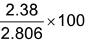= 84.82
Hence, the percentage of oxygen in the oxide

= 100.00 – 84.82 = 15.18

Step 2: To calculate the empirical formula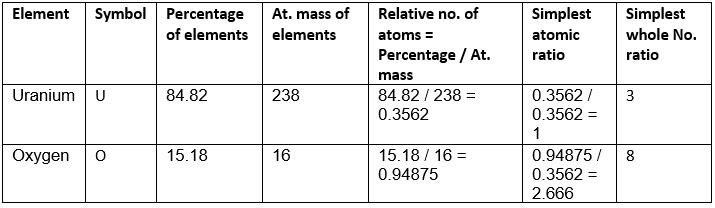Hence the empirical formula of the oxide is U3O8.

Example.5. Chemical analysis of a carbon compound gave the following percentage composition by weight of the elements present. Carbon 10.06%, hydrogen 0.84%, chlorine 89.10%. Calculate the empirical formula of the compound.
Solution. Step 1: Percentage of the elements present
Carbon = 10.06, Hydrogen = 0.84, Chlorine = 89.10.
Step 2: Dividing the percentage compositions by the respective atomic weights of the elements
10.06 / 12 = 0.84, 0.84 / 1 = 0.84, 89.10 / 35.5 = 2.51.
Step 3: Dividing each value in step 2 by the smallest number among them to get simple atomic ratio
0.84 / 0.84, 0.84 / 0.84, 2.51 / 0.84.
Step 4: Ratio of the atoms present in the molecule C : H : Cl = 1 : 1 : 3
∴ The empirical formula of the compound C1H1Cl3 or CHCl3.

Example.6. A carbon compound on analysis gave the following percentage composition. Carbon 14.5%, hydrogen 1.8%, chlorine 64.46%, oxygen 19.24%. Calculate the empirical formula of the compound.
Solution. Step1: Percentage composition of the elements present in the compound.

C : H : Cl : O = 14.5 : 1.8 : 64.46 : 19.24.

Step 2: Dividing by the respective atomic weights.
14.5 / 12 : 1.8 / 1 : 64.46 / 35.5 : 19.24 / 16 = 1.21 : 1.8 : 1.81 : 1.2.
Step 3: Dividing the values in step 2 among them by the smallest number.
1.21 / 1.21, 1.8 / 1.2, 1.81 / 1.2, 1.2 / 1.2.
Step 4: Multiplication by a suitable integer to get whole number ratio.
(1 x 2) = 2, (1.5 x 2) = 3, (1.5 x 2) = 3, (1 x 2) = 2

∴ The simplest ratio of the atoms of different elements in the compound.

C : H : Cl : O = 2 : 3 : 3 : 2
Empirical formula of the compound C2H3Cl3O2.

Example.7.The empirical formula of a compound is . Its molecular weight is 90. Calculate the molecular formula of the compound. (Atomic weights C = 12, H = 1, O = 16).
Solution. Empirical formula = CH2O
Empirical formula weight = (12 + 2 + 16) = 30
n = molecular weight / empirical formula weight
∴ n = 90 / 30 = 3.

The molecular formula (CH2O)3 = C3H6O3
Relation between the two: Molecular formula= Empirical formula × n
Check out the importance of each step involved in calculations of empirical formula.
Density
(a) Absolute density
(b) Relative density
Absolute density = Mass / Volume
Relative density = Density of substance / Density of standard substance
Specific gravity = Density of substance / Density of H2O at 4°C
Vapor density: It is defined only for gas.
It is a density of gas with respect to H2 gas at same temp & pressure.
V.D =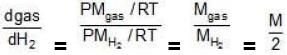V.D = M / 2
V.D = Molecular wt of gas / Molecular wt of H2 gas
Density of Cl2 gas with respect to O2 gas = Molecular wt of Cl2 gas / Molecular wt of O2 gas

TRY YOURSELF

Q.1. A crystalline hydrated salt, on being rendered anhydrous, loses 45.6% of its mass. The percentage composition of the anhydrous salt is: Al = 10.5%, K = 15.1%, S =24.8% and oxygen = 49.6%. Find the empirical formula of the anhydrous and crystalline hydrated salt.[K = 39; Al = 27; S = 32; O = 16; H = 1].
Ans. Empirical formula of anhydrous salt = KAS2O8 Hydrated salt composition; % anhydrous
part = 54.4% and % H2O = 45.6%; Empirical formula of hydrated salt = KAIS2O8.12H2O.

Q.2. A colourless crystalline compound has the following percentage composition: Sulphur 24.24%, nitrogen 21.21%, hydrogen 6.06% and the rest is oxygen. Determine the empirical formula of the compound. If the molecular mass is 132, what is the molecular formula of the compound? Name the compound if it is found to be sulphite.
Ans.
Empirical formula SN2H8O4; Molecular formula = SN2H8O4; Name – Ammonium sulphite (NH4)2SO3.

Q.3. A gaseous hydrocarbon contains 85.7% carbon and 14.3% hydrogen. 1 litre of the hydrocarbon weighs 1.26 g at NTP. Determine the molecular formula of the hydrocarbon.
Ans. C2H4.

Offer running on EduRev: Apply code STAYHOME200 to get INR 200 off on our premium plan EduRev Infinity!

,

,

,

,

,

,

,

,

,

,

,

,

,

,

,

,

,

,

,

,

,

;